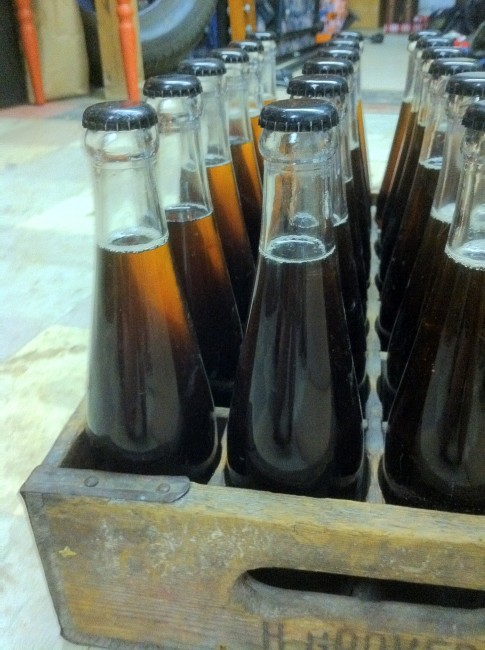## Evil Twin (ish) Red

Monday, February 28th, 2011

Last week, we brewed up the Cream Ale and used a some yeast culture (WLP 001)Â  that had been sitting in my fridge for over a year. Regardless of the age, fermentation started out strong and smelled clean.

So to reward these awesome yeasties, I decided to dump on another batch of beer once the Cream Ale was racked to secondary.

### Red RecipeThis is my first red, so I looked around and found some good examples. In the end, I decided to use a recipe modified from Jamil Zainasheff’s Evil Twin Red.

This is for a full 12gallon batch:

• 24 lb â€“ pale 2 row
• 3 lb â€“ Caramel 80
• 2 lb â€“ Munic
• 1 lb â€“ Victory
• 0.5 lb – Light Chocolate
• 3 lb – Malted Oats
• 2 oz – Millennium (pellets) @ 45 min
• 1 oz – Centennial @ 20 min
• 2 oz – Centennial @ 10 min
• 1 oz – Centennial @ 0 min
• WLP001 California Ale Yeast

Mashed at 154Â° F for 60+ minutes. Gather a pre-boil volumeÂ  of “only” 13.7 gallons. Boil for 60 minutes.

Should give us drinkable 5%ABV with 60ish IBUs.

May need to be dry hopped.

`^Evil Twin (ish) Red^^101^80^12^60^10^4^50^100^8.8^0.3^0^0^0^0^71^71^24^35^35^3^63^63^2^91^90^1^0^0^0^40^40^0.5^67^67^3^0^0^0^0^0^47^2^45^0^1^6^1^20^1^2^6^2^10^1^2^6^1^0^1^2^0^0^0^1^2^0^0^0^1^2^2^47^154^0.8^^^^More reasonable - needs some dry hop and oak^^^^^^^^^^^^^^^^^^^^14^10^10^10^0^0^0.2^`

### 3 Responses to “Evil Twin (ish) Red”

1.nagmay Says:

Ended up dry hopping with 2oz of Palisades pellets (1oz in each carboy).

2.nagmay Says:

Just got around to bottling last night. Despite almost 2 weeks of dry hopping, the hop aroma was smooth and subtle.

Half the batch was put in the clear bottles shown above.

3.nagmay Says:

Oh, crap! I just realized that my calculations were set at 50% efficiency! They should have been at 75-80.

This was an 8% beer.

No wonder it tasted so astringent!

You know you want to...